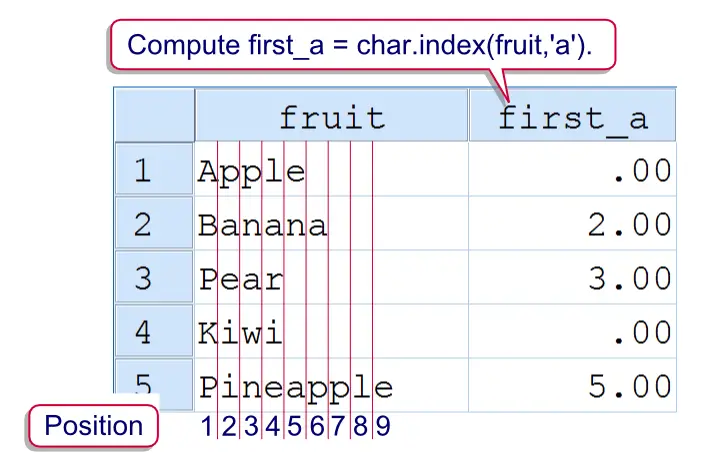SPSS TUTORIALS BASICS ANOVA REGRESSION FACTOR CORRELATION

# SPSS INDEX Function

The SPSS `INDEX` function returns the position of the first occurrence of a given expression within a string. If the expression does not occur in the string, it returns a zero. As a rule of thumb, always use it as `CHAR.INDEX`. The reason for this is explained SPSS Unicode Mode. Note that string values are case sensitive.SPSS Index Function Example

## SPSS Index Example

Say we have data holding some email addresses and we'd like to see which domains are used most. For each email address, the domain is everything after the `@` sign. The syntax below demonstrates how to do so. We'll first find the position of the first (and only)`@` in step 2. Next, we'll substitute that into a `SUBSTR` function in step 4.

## SPSS Index Syntax Example 1

*1. Create data.

data list free/email (a20).
begin data
[email protected] annek[email protected] [email protected] [email protected] maarten1979bkb.nl
end data.

*2. Find position of first "@".

compute first_a = char.index(email,'@').
exe.

*3. Declare new string variable for domain.

string domain(a15).

*4. Extract domain from email address.

compute domain = char.substr(email,char.index(email,'@') + 1).
exe.

*5. Correction for email without "@".

if char.index(email,'@') = 0 domain = ''.
exe.

Note that there's an error in the data since the last email address doesn't contain any `@`. Therefore, `first_@` is zero for this case. This makes step 4 come up with an incorrect `domain`, hence the correction at the end.A better option here is to use a single IF command that computes the domain only if `@` is present in the email address.

## SPSS Index - the Divisor

A little known feature of SPSS' `INDEX` function is an optional third argument known as the divisor. The divisor divides the search expression into substrings of length n. The position of the first occurrence of one of these substrings is returned. For example, in `CHAR.INDEX(variable,'0123456789',1)` the divisor is `1`. This breaks `0123456789` into substrings of length 1, rendering the digits `0` through `9`. The position of the first digit is now returned.

The next syntax example extracts all digits from a string. It combines the use of the divisor with LOOP, SUBSTR and CONCAT in order to do so. The last step uses ALTER TYPE for converting it into a numeric variable.

## SPSS Index Syntax Example 2

*1. Check whether any number is present in email.

compute number_present = char.index(email,'0123456789',1) > 0.
exe.

*2. Declare new string.

string numbers(a20).

*3. Loop through characters and pass each digit into string.

loop #pos =1 to char.length(email).
if char.index(char.substr(email,#pos,1),'0123456789',1) > 0 numbers = concat(numbers,char.substr(email,#pos,1)).
end loop.
exe.

*4. Convert string to numeric variable.

alter type numbers(f1.0).

# Tell us what you think!

*Required field. Your comment will show up after approval from a moderator.